## Introduction

Recent work has suggested the use of metalenses for broadband achromatic focusing1. Here, we show that it is not necessary to invoke concepts of metasurfaces or metalenses to enable such focusing. Scalar diffractive optics, when designed appropriately, can readily enable ultra-broadband achromatic focusing. Such diffractive optics can be far simpler to manufacture and can allow for polarization-independent focusing. An ideal lens focuses one point in the object space to one point in the image space2. Almost all imaging systems suffer from chromatic aberrations, which means that light of different wavelengths generate focal spots at different spatial locations2. This phenomenon deteriorates the performance of both imaging3,4 and non-imaging5 systems under broadband illumination. For instance, a color camera without chromatic-aberration correction will form spatially displaced and defocused images of the blue, green and red channels.

Chromatic aberration is due to either the dispersion properties of the material or the structure of the optic. For refractive lenses, longer wavelengths focus at a farther distance, since in most dielectric materials, the refractive index decreases at longer wavelengths. Figure 1(a) illustrates the simple example of a bi-convex glass lens and the corresponding shift of its focus, calculated by the Lensmaker’s equation2. The conventional diffractive lens (zone-plate), on the other hand, exhibits opposite chromatic aberration (Fig. 1(b))6,7,8. Diffraction angle is proportional to wavelength2 and thus longer wavelengths are focused closer than shorter ones.

Chromatic aberration can be corrected approximately by using materials that exhibit complementary dispersion, as in an achromatic doublet and triplet9,10,11. However, this technique is cumbersome, since the number of materials equals the number of wavelengths where the chromatic aberrations are minimized10,11. The extra alignment makes these lenses expensive and bulky. Hybrid refractive-diffractive lenses perform slightly better, but their complexity is even higher12,13,14. Such designs that work for more than three wavelengths are seldom studied. An alternative approach is to use a phase-coded aperture15, but this requires precise polishing of the glass surface. In all these cases, it is challenging to make such corrected lenses with micro-scale thickness.

Metasurfaces exploit surface plasmonic or nanophotonic phenomena to locally impart abrupt phase shift so as to purposely manipulate the diffraction pattern16,17. Previous studies showed its potential in anomalous reflection and refraction and complex beam generation16,18,19. Here, we emphasize that metasurfaces are excellent, when the vector properties of light must be manipulated as in the case of a high-efficiency polarizer20, but they are not required to manipulate the scalar properties of light. Diffractive optics is a better alternative. The fabrication requirements for metasurfaces are far more stringent in terms of both resolution and precision compared to diffractive optics. Furthermore, metasurfaces are by nature polarization sensitive1,16,17,18,19,20. Here, we reiterate that diffractive optics can readily enable broadband focusing, while still maintaining the planar architecture. Previously, we have functionalized diffractive optics as a solar spectrum splitter/concentrator21, multi-color encoder22, phase masks for 3D lithography23 and dispersion elements in computational spectroscopy24.

Here, we extend the concept of broadband diffractive optics to super-achromatic focusing. Specifically, we designed, fabricated and characterized 4 different planar cylindrical chromatic-aberration-corrected lenses. Each lens has a maximum thickness of 3 μm and a minimum feature size of 3 μm. All the devices can be readily patterned using grayscale lithography and inexpensively replicated for mass-production using imprint lithography25,26. The aberration-correction capabilities of our lenses are on par with or better than commercial doublets. Two types of lenses were constructed. One was designed for three discrete wavelengths and the other for continuous broadband illumination.

The cross-sectional schematic of our chromatic-aberration-corrected diffractive lens (CACDL) is illustrated in Fig. 2(a). The CACDL is composed of pixels that can be square (2D) or linear grooves (1D). In the devices described here, the grooves are of width, Δ = 3 μm and height, hi is assigned to the ith groove. Each groove imparts a relative phase shift given by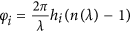, where n(λ) is the refractive index of device material at wavelength λ22. For simplicity, we utilize a positive-tone photoresist, SC1827 deposited on a soda-lime glass substrate as the device material. A commercial grayscale lithography tool was employed to rapidly pattern the device in a single step21,22,23,24. The width, Δ is dictated by the resolution of this tool.

In order to achieve super-achromatic performance, we applied a modified direct-binary-search (DBS) algorithm to optimize the distribution of groove heights, hi21,22,23,24,27. It is a perturbation-based iterative method. Details of the design algorithm are included in the Supplementary Materials. The target point-spread function (PSF) is defined as a diffraction-limited Gaussian with full-width-at-half-maximum (FWHM) determined by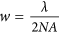. The numerical aperture (NA) is given by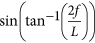in which L = NΔ is the total length of the lens, N is the total number of grooves and f is the design focal length. Compared to other optimization algorithms for multi-wavelength diffractive optics28,29, our technique is applicable generally20,21,22,23,24 and our approach is the first experimental demonstration of super-achromatic and continuous broadband focusing using diffractive optics.

The diffraction pattern in the focal plane is determined by the phase acquired by light transmitting the diffractive lens (Fig. 2(c)) and that acquired via the optical path length in air (Fig. 2(b)). For chromatic-aberration-corrected focusing at x′, three or more wavelengths must diffract from location, x such that they interfere constructively at the focus, x′. Our method for designing the diffractive lens realizes an optimal height distribution that can approximate such constructive interference. In our lens, there are N grooves and each groove can occupy P discrete height levels. Therefore, the total degrees-of-freedom can be enormous, PN. This allows extreme design flexibility as illustrated later. We designed and fabricated four CACDLs (see Fig. 2(d)). For each device, we assumed periodic boundaries during design and fabricated 7 periods, each of length L = 8.4 mm. The optical micrographs, profilometer measurements of the topography and the scanning-electron micrographs of exemplary devices are shown in Fig. 2(e,f,g), respectively. The cross-sectional micrographs of a cleaved sample (Fig. 2(g)) indicate that the grooves are rounded due to the resolution limitations of our lithography tool. Nevertheless, the average height within each groove was within 100 nm of the design value. It is noteworthy that the maximum aspect ratio is 1:1, which is much smaller than that of the metalenses1,30. Furthermore, the lithographic resolution required for our CACDLs is only 3 μm (5λ for λ = 600 nm) compared to ~100 nm (0.065λ for λ = 1550 nm) for the metalenses1. To achieve broadband focusing in the visible region with the metalenses, one would require features of size 39 nm and aspect ratios of over 3:1.

To experimentally demonstrate chromatic-aberration-corrected focusing, we illuminated each CACDL using a spatially collimated beam from a super-continuum source (SuperK EXTREME EXW6, NKT Photonics) that was first conditioned using a reconfigurable band-pass filter (SuperK VARIA, NKT Photonics). The filter allowed us to illuminate the CACDL with one discrete wavelength at a time (minimum bandwidth 10 nm). Then, a single-mode fiber (SMF, core diameter ~8 μm) connected to a spectrometer (Ocean Optics Jaz) was placed in the vicinity of the designed focus. The fiber was scanned using a motorized 2-axis stage with 3 μm and 10 μm steps along the X′ and Z axes, respectively. The transmitted spectra were collected at each location. The final results were derived after subtracting the dark spectrum from the raw data and dividing by the reference spectrum (that transmitted through the unpatterned photoresist).

First, we consider the CACDLs designed for three discrete wavelengths (460 nm nm, 540 nm and 620 nm). To demonstrate the flexibility of our approach, we designed 3 different lenses with the following parameters: number of grooves, N = 2800, 2800, 280; focal length, f = 120 mm, 25 mm and 10 mm, which correspond to numerical aperture, NA = 0.035, 0.166 and 0.042, respectively. Figure 3(a–i) summarize the simulated and measured light-intensity distributions in the focal plane at the 3 design wavelengths. As expected all 3 lenses exhibit clear apochromatic focusing. Scalar-diffraction simulations predict average optical efficiencies of 30.0%, 30.4% and 39.0% for the 3 designs. The corresponding measured average optical efficiencies are 24.9%, 23.0% and 21.5%, much higher than those of previously reported achromatic lenses13. Even higher efficiency (>50%) is possible with thicker microstructures (Fig. S13 in the Supplementary Materials). In theory, non-ideal efficiency (<100%) is primarily due to lack of perfect interference (constructive at focus and destructive in the background). Generally speaking, this efficiency dictates the contrast or resolution in an optical system. Here, we define the optical efficiency as the ratio of power within the region defined by the first zero to the total incident power. We can also quantify the achromaticity of the CACDLs by measuring the lateral and axial focus shifts as a function of wavelength. These can be calculated by comparing the 2D PSF (XZ plane) at each wavelength to that at the center wavelength, 540 nm. The lateral and axial focus shifts for the first design were 0.32 μm, 6.7 μm (simulation) and 1.3 μm, 25 μm (experiment), respectively. These are better than what can be achieved using conventional refractive lens combinations9.

Due to the finite diameter of the SMF core, the measured PSFs are wider than the actual distributions. This is especially obvious in the CACDL with the highest NA (Fig. 3(d–f)). Fabrication errors as well as the limited acceptance angle of the SMF contribute to the reduction of optical efficiencies. The 2D PSFs (XZ) of the first design at five wavelengths (460 nm, 500 nm, 540 nm, 580 nm and 620 nm) are plotted in Fig. 3(j–n) (simulation) and Fig. 3(o–s) (measurement). The scalar-diffraction simulation has resolution of 0.2 μm and 2.5 μm in X′ and Z directions. The measured plots are numerically interpolated into the same grid for visual comparison. At the vicinity of the nominal focal plane (white-dashed lines), focusing is clearly observed for only the design wavelengths (460 nm, 540 nm and 620 nm). No focusing is found at the other wavelengths (Fig. 3(k,p,m,r)). Another simple evidence of apochromatic focusing is seen via the images captured at the focal plane using a monochrome sensor (DMM22BUC03-ML, The Imaging Source) with illumination wavelength selected by the VARIA filter, shown as insets in Fig. 3(o–s). Note that the SMF-spectrometer scheme was used to accurately measure PSFs (Fig. 3(a–i) and (o–s)), since the spectrometer has higher spectral resolution (0.4 nm) than the VARIA filter and larger dynamic range (16-bit) than the sensor (8-bit).

Next, we extended our CACDL to focus continuous broadband illumination across the visible spectrum (450 nm–690 nm, super-achromatic). This is achieved by increasing the wavelength sampling to 5 nm during design. It was designed with N = 2500, focal length, f = 280 mm and NA = 0.013. The simulated and measured 1D PSFs in the design focal plane as a function of wavelength are plotted in Fig. 4(a,b), respectively. Note that the plots are normalized to the peak at each wavelength to account for the spectrum of the source. The white dots (left) and crosses (right) indicate the lateral (Δx) and axial (Δf ) focal-spot shifts in each figure. These shifts were obtained from the 2D (XZ) PSFs. The simulated and measured 2D PSFs at 3 wavelengths are illustrated in Fig. 4(d–f) and 4(g–i), respectively. Again, the measurements were interpolated into the same resolution as the simulations. The lateral shift averaged over all wavelengths,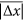is 0.47 μm (simulation) and 1.65 μm (experiment). The axial shift averaged over all wavelengths,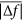is 23.5 μm (simulation) and 73.6 μm (experiment). Both shifts are significantly smaller than that of a diffractive lens optimized for single wavelength (Fig. S12 in the Supplementary Materials). The maximum axial-focus shift, Δf is comparable to that of commercial achromatic doublets9. However, our CACDL is thin (planar), inexpensive and comprised of only a single material. The optical-efficiency spectrum is plotted in Fig. 4(c). The discrepancies between the simulated and measured curves are primarily due to fabrication errors in the CACDL height profile. The efficiency drops at longer wavelengths. This can be potentially compensated by appropriately weighting the efficiencies of different wavelengths during design21 and by optimizing the patterning process. As before, monochrome images illuminated by the 3 wavelengths (selected by the VARIA) are shown as insets in Fig. 4(g–i).

In all micro-optics, fabrication errors pose an important impact on the optical efficiencies. We numerically analyzed this impact by adding random errors with various standard deviations to the design-height distribution. The results plotted in Fig. 5(e) indicate that the CACDLs are robust to height errors of up to ~100 nm, which, in turn, corresponds to two height levels (Δh = H/(P−1) = 50 nm). Therefore, our device is relatively tolerant to fabrication errors, which is consistent with previous devices designed using related techniques20,21,22,23,24. As expected, the efficiency decreases with increasing errors (left Y axis in Fig. 5(e)) and the device with fewer grooves (CACDL#3) is more susceptible to fabrication errors21,22. This is because constructive interference gradually breaks down when the phase distribution deviates from the optimal design. Moreover, the wavelength-averaged axial-focus shift,increases with errors (right Y axis in Fig. 5(e)). For CACDL#1,is maintained small when the error is less than 100 nm, while that of the CACDL#4, it deteriorates rapidly. This is likely a consequence of the fact that broadband super-achromatic focusing requires a more stringent phase matching compared to the case of focusing only 3 wavelengths.
We also simulated the impact of oblique incidence (Fig. 5(f)). The wavelength-averaged focus shifts both laterally and axially with change in incident angle, θ. Hence, the wavelength-averaged optical efficiency drops with off-normal incidence (top panel). Nevertheless, both studied CACDLs maintain their efficiencies over θ~±4o. The wavelength-averaged lateral-focus shift,(middle panel) and axial-focus shift,(bottom panel) increase nonlinearly with θ. However, both designs preserve reasonable chromatic aberrations over θ ~ ±4o. Note that even though we assumed periodic boundaries during design, experiments suggest that this is not strictly necessary as elaborated in the Supplementary Materials. Finally, although our devices were 1D, they can be readily extended to 2D20,22,23 and also to almost any electromagnetic spectrum.